# ISEE Middle Level Math : How to find the whole from the part with percentage

## Example Questions

2 Next →

### Example Question #180 : Percentage

At a pet store, there are 12 dogs and 8 cats. The following week, the number of dogs increases by 25%, while the number of cats decreases by 25%. What is the total number of animals at the store the following week?

Assume the store only sells dogs and cats.Explanation:

If the population of dogs increases by 25%, then we first find 25% of 12 (which is 3) and add the 3 to 12. This gives us 15 dogs.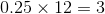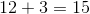If the population of cats decreases by 25%, then we find 25% of 8 (which is 2), and subtract if from 8. This gives us 6.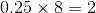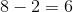Now that we know the final individual numbers of dogs and cats, we can add them to find the final answer.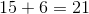### Example Question #11 : How To Find The Whole From The Part With Percentage

Tom the baker madeblueberry muffins on Tuesday, and Stan the baker made andmuffins that day. On Wednesday, each baker decided to increase his production of blueberry muffins by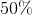. What is the average of blueberry muffins that Tom and Stan baked on Wednesday?Explanation:

Tom madeblueberry muffins on Tuesday, and Stan made andmuffins that day. On Wednesday, each baker decided to increase his production of blueberry muffins by.

Given thatofis, the number of muffins made by Tom on Wednesday would be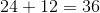Given thatofis, the number of muffins made by Stan on Wednesday would be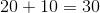The average is calculated by adding numbers in a list together, and then dividing by the number of items in that list. This gives us: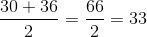### Example Question #12 : How To Find The Whole From The Part With Percentage

10 percent of a cake weights 20 ounces. The entire cake is cut into 8 pieces. How many ounces does each piece weigh?ouncesouncesouncesouncesounces

Explanation:

If 10 percent of the cake weights 20 ounces, then the entire cake will weigh 200 ounces, because 10 times 20 is 200.

If the cake has 8 slices, then each slice will weigh (in ounces) 200 divided by 8. 200 divided by 8 is 25; therefore, 25 ounces is the correct answer.

### Example Question #13 : How To Find The Whole From The Part With Percentage

There are 12 sopranos in the Red Street Choir.  If sopranos are 40% of the choir, how many total members make up the Red Street Choir?Explanation:

When given a part, you can use a percentage to determine the whole by converting the percentage to a decimal and dividing the part by that number.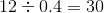### Example Question #14 : How To Find The Whole From The Part With Percentage

If you have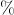of a quiz completed afterquestions, how long is the quiz?Explanation:

To find a part of a whole with a percentage, you multiply the whole by the percent.

To find the opposite, you divide the part by the percent so it would be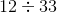.

This is equal to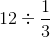or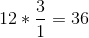.

### Example Question #15 : How To Find The Whole From The Part With Percentage

There are 8 pieces of pizza. If Justin at 25% of the pizza, how many pieces did he have?Explanation:

25% is equivalent to the fraction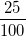and can then be reduced to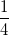which is equivalent to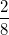,

meaning that if Justin ate 25% of the pizza, he ate 2 of the 8 pieces.

2 Next →